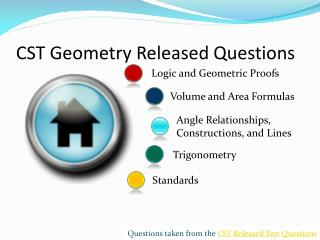DownloadDownload PresentationCST Geometry Released Questions

# CST Geometry Released Questions

Download Presentation## CST Geometry Released Questions

- - - - - - - - - - - - - - - - - - - - - - - - - - - E N D - - - - - - - - - - - - - - - - - - - - - - - - - - -
##### Presentation Transcript

1. CST Geometry Released Questions Logic and Geometric Proofs Volume and Area Formulas Angle Relationships, Constructions, and Lines Trigonometry Standards Questions taken from the CST Released Test Questions

2. Geometry 1

3. Geometry 1

4. Geometry 1

5. Geometry 2

6. Geometry 2

7. Geometry 2

8. Geometry 2

9. Geometry 3

10. Geometry 3

11. Geometry 3

12. Geometry 3

13. Geometry 3

14. Geometry 4

15. Geometry 4

16. Geometry 4

17. Geometry 4

18. Geometry 4

19. Geometry 4

20. Geometry 4

21. Geometry 4

22. Geometry 5

23. Geometry 5

24. Geometry 5

25. Geometry 6

26. Geometry 6

27. Geometry 7

28. Geometry 7

29. Geometry 7

30. Geometry 7

31. Geometry 7

32. Geometry 7

33. Geometry 7

34. Geometry 8

35. Geometry 8

36. Geometry 8

37. Geometry 8

38. Geometry 8

39. Geometry 9

40. Geometry 9

41. Geometry 9

42. Geometry 10

43. Geometry 10

44. Geometry 10

45. Geometry 10

46. Geometry 10

47. Geometry 10

48. Geometry 10

49. Geometry 11

50. Geometry 11